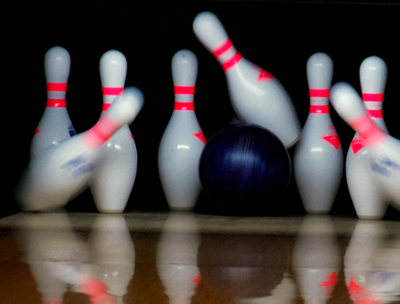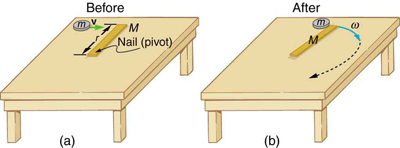# 10.6 Collisions of extended bodies in two dimensions

 Page 1 / 3
• Observe collisions of extended bodies in two dimensions.
• Examine collision at the point of percussion.

Bowling pins are sent flying and spinning when hit by a bowling ball—angular momentum as well as linear momentum and energy have been imparted to the pins. (See [link] ). Many collisions involve angular momentum. Cars, for example, may spin and collide on ice or a wet surface. Baseball pitchers throw curves by putting spin on the baseball. A tennis player can put a lot of top spin on the tennis ball which causes it to dive down onto the court once it crosses the net. We now take a brief look at what happens when objects that can rotate collide.

Consider the relatively simple collision shown in [link] , in which a disk strikes and adheres to an initially motionless stick nailed at one end to a frictionless surface. After the collision, the two rotate about the nail. There is an unbalanced external force on the system at the nail. This force exerts no torque because its lever arm $r$ is zero. Angular momentum is therefore conserved in the collision. Kinetic energy is not conserved, because the collision is inelastic. It is possible that momentum is not conserved either because the force at the nail may have a component in the direction of the disk’s initial velocity. Let us examine a case of rotation in a collision in [link] .The bowling ball causes the pins to fly, some of them spinning violently. (credit: Tinou Bao, Flickr)(a) A disk slides toward a motionless stick on a frictionless surface. (b) The disk hits the stick at one end and adheres to it, and they rotate together, pivoting around the nail. Angular momentum is conserved for this inelastic collision because the surface is frictionless and the unbalanced external force at the nail exerts no torque.

## Rotation in a collision

Suppose the disk in [link] has a mass of 50.0 g and an initial velocity of 30.0 m/s when it strikes the stick that is 1.20 m long and 2.00 kg.

(a) What is the angular velocity of the two after the collision?

(b) What is the kinetic energy before and after the collision?

(c) What is the total linear momentum before and after the collision?

Strategy for (a)

We can answer the first question using conservation of angular momentum as noted. Because angular momentum is $\mathrm{I\omega }$ , we can solve for angular velocity.

Solution for (a)

Conservation of angular momentum states

$L=L\prime ,$

where primed quantities stand for conditions after the collision and both momenta are calculated relative to the pivot point. The initial angular momentum of the system of stick-disk is that of the disk just before it strikes the stick. That is,

$L=\mathrm{I\omega },$

where $I$ is the moment of inertia of the disk and $\omega$ is its angular velocity around the pivot point. Now, $I={\text{mr}}^{\mathrm{\text{2}}}$ (taking the disk to be approximately a point mass) and $\omega =v/r$ , so that

$L={\text{mr}}^{2}\frac{v}{r}=\text{mvr}.$

After the collision,

$L\prime =I\prime \omega \prime .$

It is $\omega \prime$ that we wish to find. Conservation of angular momentum gives

$I\prime \omega \prime =\text{mvr}.$

Rearranging the equation yields

$\omega \prime =\frac{\text{mvr}}{I\prime },$

where $I\prime$ is the moment of inertia of the stick and disk stuck together, which is the sum of their individual moments of inertia about the nail. [link] gives the formula for a rod rotating around one end to be $I={\text{Mr}}^{2}/3$ . Thus,

#### Questions & Answers

what there factors affect the surface tension of a liquid
formula for impedance
ehat is central forces
what is distance?
What does mean ohms law imply
ohms law state that the electricity passing through a metallic conductor is directly proportional to the potential difference across its end
muyiwa
what is matter
Anything that occupies space
Kevin
Any thing that has weight and occupies space
Victoria
Anything which we can feel by any of our 5 sense organs
Suraj
Right
Roben
thanks
Suraj
what is a sulphate
Alo
Alo
the time rate of increase in velocity is called
acceleration
Emma
What is uniform velocity
Victoria
Greetings,users of that wonderful app.
how to solve pressure?
how do we calculate weight and eara eg an elefant that weight 2000kg has four fits or legs search of surface eara is 0.1m2(1metre square) incontact with the ground=10m2(g =10m2)
Cruz
P=F/A
Mira
can someone derive the formula a little bit deeper?
Bern
what is coplanar force?
forces acting and lying on d same plane
Promise
what is accuracy and precision
How does a current follow?
follow?
akif
which one dc or ac current.
akif
how does a current following?
Vineeta
?
akif
AC current
Vineeta
AC current follows due to changing electric field and magnetic field.
akif
you guys are just saying follow is flow not follow please
Abubakar
ok bro thanks
akif
flows
Abubakar
but i wanted to understand him/her in his own language
akif
but I think the statement is written in English not any other language
Abubakar
my mean that in which form he/she written this,will understand better in this form, i write.
akif
ok
Abubakar
ok thanks bro. my mistake
Vineeta
u are welcome
Abubakar
what is a semiconductor
substances having lower forbidden gap between valence band and conduction band
akif
what is a conductor?
Vineeta
replace lower by higher only
akif
convert 56°c to kelvin
Abubakar
How does a current follow?
Vineeta
A semiconductor is any material whose conduction lies between that of a conductor and an insulator.
AKOWUAH
what is Atom? what is molecules? what is ions?
atoms are the smallest unit of an element which is capable of behaving as a single unit
Promise
a molecule is d smallest unit of a substances capable of independent existence and can also retain the chemical proper ties of that substance
Promise
an ion is referred to as freely moving charged particles
Promise
What is a molecule
Is a unit of a compound that has two or more atoms either of the same or different atoms
Justice
A molecule is the smallest indivisible unit of a compound, Just like the atom is the smallest indivisible unit of an element.
Rachel
what is a molecule?
VineetaByByByByByByByByBy